# Logical Operators

A logical operator compares Boolean operands and returns either True or False (a Boolean result). Table 10.2 presents a list of the logical operators that Visual Basic .NET makes available.

##### Table 10.2. The Visual Basic .NET Logical Operators

Logical Operator

Type

Meaning

And

Binary

Logical And

`  Or  `

Binary

Logical Or

Xor

Binary

Logical exclusive Or

Not

Unary

Logical negation

AndAlso

Binary

Short-circuit And

OrElse

Binary

Short-circuit Or

As you can see in Table 10.2, all but logical Not are binary operators. The following sections explore each of these logical operators in detail.

#### The Logical And Operator

The logical And operator compares two operands and returns logic True if, and only if, both operands are logic True . You can see this relationship by looking at the truth table for the logic And operator. A truth table is a table that shows a list of all possible combinations for the two operands and their logical And result. Table 10.3 presents the truth table for logical And .

##### Table 10.3. The Truth Table for the Logical And Operator

Operand1

Operand2

Result

True

True

True

True

False

False

False

True

False

False

False

False

Notice in Table 10.3 that the only way to get a True result with a logical And operator is when both operands are True . Any other combination produces a False result. Consider the following expression:

` Result = 10 > 5 And 100 > 60 `

If you evaluate each relational test in this expression, you get this:

` Result = 10 > 5 And 100 > 60  Result = True And True  ' Cols 1 and 2 in Row 1,Table 10.3 Result = True      ' Row 1, Column 3 in Table 10.3 `

Because 10 is greater than 5 , the first relational test is True . Also, 100 is greater than 60 , so the second relational test is also True . Therefore, the second line of this example shows that the logical test that is actually being performed is True And True . From Table 10.3, because both operands are True , the expression is True .

Let's try a variation on the same expression but change the relational operator for the second operand:

` Result = 10 > 5 And 100 < 60  Result = True And False  ' Cols 1 and 2 in Row 3,Table 10.3 Result = False      ' Row 2, Column 3 in Table 10.3 `

Here are some additional examples, with the results shown in the comments at the ends of the statements:

` Result = 10 = 5 And 100 > 60        ' False  Result = 10 >= 5 And 100 <> 60      ' True Result = 10 <> 5 And 100 <> 60      ' True Result = 10 > 5 And 100 <= 60        ' False Result = 10 > 5 And 100 > 60 And 20 < 80  ' True Result = 10 > 5 And 100 < 60 And 20 < 80  ' False `

Work through each example and convince yourself that the result is as it indicated. The last two statements show that you can have more than just one logical And operator in a single statement.

#### The Logical Or Operator

The logical Or operator compares two operands and returns a logic True if either of the operands is logic True . The truth table for the logical Or operator is shown in Table 10.4.

##### Table 10.4. The Truth Table for the Logical Or Operator

Operand1

Operand2

Result

True

True

True

True

False

True

False

True

True

False

False

False

A logical Or test produces a True result any time either of the operands is True . Only when both operands are False does the logical Or test produce a False result. Let's use the same examples as before, but test them using the logical Or operator:

` Result = 10 = 5 Or 100 > 60        ' True  Result = 10 >= 5 Or 100 <> 60        ' True Result = 10 <> 5 Or 100 <> 60        ' True Result = 10 > 5 Or 100 <= 60        ' True Result = 10 > 5 Or 100 > 60 Or 20 < 80    ' True Result = 10 > 5 Or 100 < 60 Or 20 < 80    ' True `

Because at least one expression using the relational operators here is True , Result is True for all the expressions. You might want to compare the results of using And and Or to make sure you understand why they are different.

#### The Logical Xor Operator

The Xor operator is the exclusive Or operator. The Xor operator returns True if one, and only one, of the operands evaluates to True . If both operands are True or both operands are False , the result is False . The truth table for the Xor operator is presented in Table 10.5.

##### Table 10.5. The Truth Table for the Logical Xor Operator

Operand1

Operand2

Result

True

True

False

True

False

True

False

True

True

False

False

False

Let's again use the same examples as before, but test them using the logical Xor operator:

` Result = 10 = 5 Xor 100 > 60          ' True  Result = 10 >= 5 Xor 100 <> 60        ' False Result = 10 <> 5 Xor 100 <> 60        ' False Result = 10 > 5 Xor 100 <= 60          ' True Result = 10 > 5 Xor 100 > 60 Xor 20 < 80    ' True Result = 10 > 5 Xor 100 < 60 Xor 20 < 80    ' False `

The first four statements are fairly easy to understand, but the last two might benefit from a little explanation. Let's work through the first of these two statements:

` Result = 10 > 5 Xor 100 > 60 Xor 20 < 80  Result = True Xor True Xor True Result = False Xor True Result = True                ' True `

The tricky part comes in step 2. Because you are evaluating an expression that has two Xor operators, the precedence levels are the same for the two operations. Therefore, step 2 actually behaves as though it were written like this:

` Result = (True Xor True) Xor True        ' True `

As you can see from Table 10.5, when both operands are True , Xor yields a False result. In the final step, False Xor True yields a True value for Result .

For the other complex statement, the resolution is as follows :

` Result = 10 > 5 Xor 100 < 60 Xor 20 < 80  Result = (True Xor False) Xor True Result = True Xor True Result = False                ' False `

This resolves to False because the final expression must resolve two True expressions.

Quite honestly, you probably won't use the Xor operator that much. However, it can be very useful in manipulating images in graphics programming.

#### Bitwise Operations Using Logical Operators

There are times when you need to get down to the bit level of a piece of data. Common examples of this need can be found in decoding of data that has been encoded, communications software, and some graphics programming. To explore the bitwise operations, we can use the code from the program shown in Figure 10.1. You need to add lines to the btnTest object's Click() event so that the procedure is written as shown in Listing 10.2. Only the top few lines are shown because the rest of the listing remains unchanged from the code shown in Listing 10.1.

##### Listing 10.2 A Partial Listing for the btnTest Object's Click() Event
` Private Sub btnTest_Click(ByVal sender As System.Object, ByVal e As _              System.EventArgs) Handles btnTest.Click  ckbTrue.Checked = False   ' Set to false to begin with  ckbFalse.Checked = False  Dim Result As Boolean    ' New code line here!  Result = Cbool(5 And 4)      ' The last new line here!  Select Case WhichOne   Case 1       ' The rest of the code for the procedure `

Notice the two new lines in Listing 10.2 that define a variable named Result as a Boolean data type. The next line simply has an And test on the values 5 and 4 . You should compile the program with the two new lines, but you shouldn't run it yet.

##### Setting a Debugger Breakpoint

With the code for the btnTest object's Click() event showing in the Form window, you need to click in the left margin of the line that has the Select Case statement. A brownish-red colored line that is similar to the one shown in Figure 10.3 should appear. This brownish-red colored line is called a breakpoint line, and it indicates that you have set a breakpoint in the program on this line.

##### Figure 10.3. Setting a breakpoint in the btnTest object's Click() event.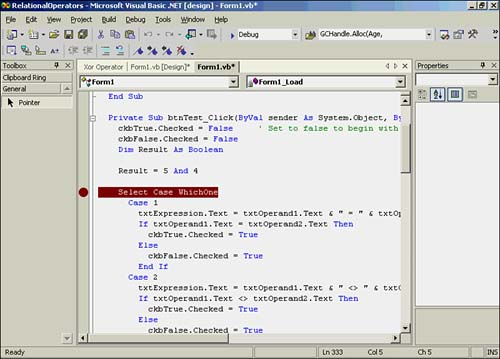A breakpoint is a feature of the Visual Basic .NET program debugger that allows you to stop program execution at the breakpoint line. When the breakpoint line is reached, Visual Basic .NET suspends program execution at the selected line. You can use a breakpoint to inspect the values of variables as they exist at any present moment during program execution.

After you have set the breakpoint and compiled the program, you should press the F5 key. (Remember that pressing the F5 key is another way of starting the program to run.) You should then click the Test button. Figure 10.4 shows the program when the breakpoint is reached.

##### Figure 10.4. Reaching a breakpoint in the btnTest object's Click() event.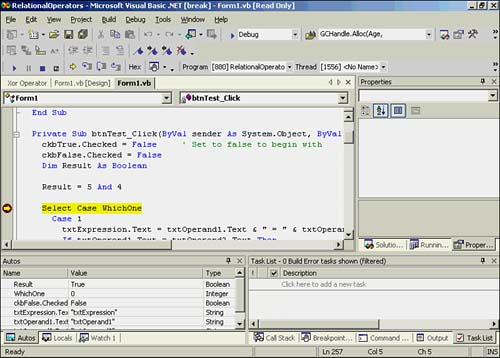Notice that the program suspends execution at the breakpoint. The brownish-red line has changed color to yellow, to indicate that the program is currently suspended at the yellow- colored line. Near the lower-left side of Figure 10.4, you see tabs that are labeled Autos, Locals, and Watch 1. Click the Locals tab. The display should look similar to Figure 10.5. (You can stop the debugger by selecting Debug, Stop Debugging or by pressing the blue square on the toolbar.)

##### Figure 10.5. Viewing the Locals window for the btnTest object's Click() event.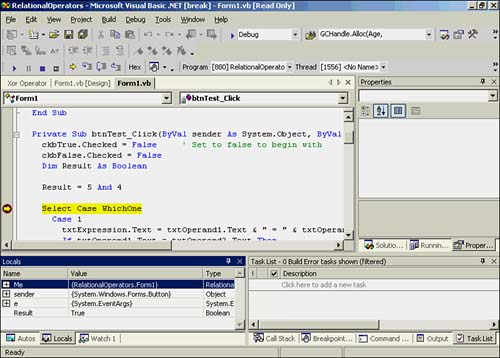As you can see in Figure 10.5, the Locals window is displaying the variables that have local scope. Among these is the Result variable, which has the value True . In other words, the logical And of 5 and 4 yields Boolean True . Why is the test True ? Because the values of 5 and 4 are nonzero, they are viewed as logic True operands, hence the True assignment to Result .

Next, you should change the definition of Result from a Boolean data type to Integer :

` Dim Result As Integer `

After you make the change, recompile and run the program. The output should look similar to that shown in Figure 10.6.

##### Figure 10.6. Result as the outcome of a bitwise And operation.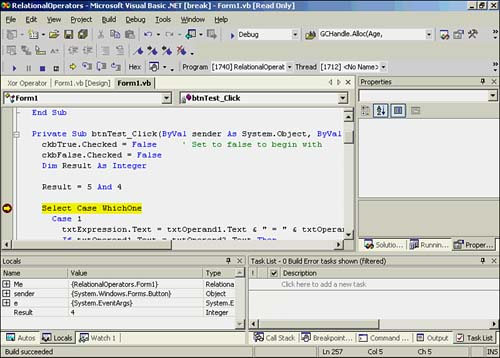Result now has the value 4 rather than True . This happens because you have defined Result as an Integer instead of a Boolean . When Result was defined as a Boolean , it could only assume the values True and False . Result is now free to hold the answer to the bitwise And of 5 and 4 . What's going on?

A bitwise And operation does a bit-by-bit comparison of the two values, logically And ing each bit in the two numbers. These are the binary representations for the two numbers :

` 5 = 00000101 4 = 00000100 `

Remember that the first bit in a number is actually the rightmost bit. For 5 , the first bit is 1 . For 4 , the first bit is . Remember from Chapter 4 that you can view a 1 as logic True for the bit and as logic False . Therefore, the first bit for 5 is True , and the first bit for 4 is False . Now look at Table 10.3. For a logical And operator, True And False yields a logic False result, or .

If you repeat this logical And for each bit of the two numbers, you get the following result:

` 5 = 00000101 4 = 00000100 ------------     00000100 = bitwise And of 5 and 4 `

Notice that only bit position 3 yields 1 from the And ing of each of the bits. This is because both bits are logic True in bit position 3. If you convert the binary result to a decimal number, the value is 4 . This is exactly what Result equals in the Locals window in Figure 10.6.

Try some different values and see what happens. Try changing the values to 16 and 4 , recompile the program, and observe the results. Here's the outcome with these values:

` 16 = 00010000  4 = 00000100 -------------      00000000 = bitwise And of 16 and 4 `

In this case, because none of the bits have matching 1s in both numbers, the result is .

##### Bitwise Or and Xor

You can use the Or and Xor functions in bitwise operations. To see this in action, change the And statement from Listing 10.2 to this:

` Result = 5 Or 4 `

Then recompile and run the program to see the result. Using Table 10.4 to generate the bitwise results, you find this:

` 5 = 00000101 4 = 00000100 ------------     00000101 = bitwise Or of 5 and 4 `

The value is 5 . This is because logical Or is True if either operand is True . What about 16 Or 4 ?

` 16 = 00010000  4 = 00000100 -------------      00010100 = bitwise And of 16 and 4 `

If you convert the binary value, you find that the value is 20 .

Now try Xor on the two numbers 5 and 4 , using Table 10.5:

` 5 = 00000101 4 = 00000100 ------------     00000001 = bitwise Xor of 5 and 4 `

Because Xor returns logic True only when the bits are different, only the first bit is True . The value is 1 . What about 16 and 4 ?

` 16 = 00010000  4 = 00000100 -------------      00010100 = bitwise Xor of 16 and 4 `

In this case, the result is again 20 .

Most programs you write will probably not use the bitwise operators. However, there are instances in communications work (for example, working with modems), image processing, and encoding “decoding algorithms in which the bitwise operations may prove useful.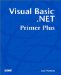Visual Basic .NET Primer Plus
ISBN: 0672324857
EAN: 2147483647
Year: 2003
Pages: 238
Authors: Jack Purdum

Similar book on Amazon